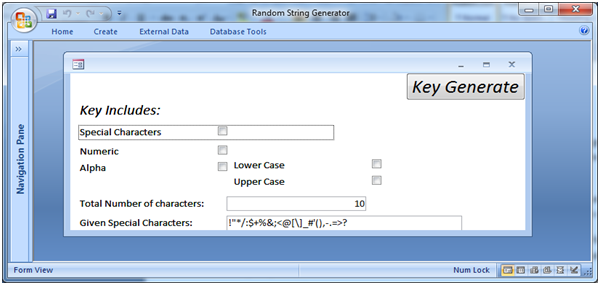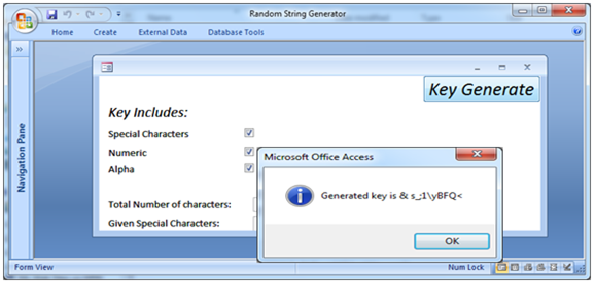# Random Key Generator

To generate random key or string, we need a string generator utility that will generate string key with specified characters. In this article we create a sample utility that will generate a key string. Using this utility user can specify the letters of its own choice.

Key generator is the part of most of MS Access VBA based application. So focusing on this, we create this utility that will very helpful for MS Access VBA programmers. In this article we explain each step visually.

For creating a Random Key generator, we need to create a form for user interface. There are different options, which user needs to specify. At least one option is required to generate the key. User can use multiple options.

Key Includes: Special Characteristics, numeric, Alphabets (Lower order or Upper order) and user can also specified the total number of characters of the key. The form contained the following controls as shown in fig 1.1.Fig:-1.1

On Key generate button we use the following VBA code that will generate the random key string as shown in Fig 1.2.Fig:-1.2

VBA code:

Option Compare Database
Option Explicit
Private Sub cmd_GenStr_Click()
Dim strGenKey As String
Dim strSQL As String
Dim i As Long
Dim intRandomStr As Integer
Dim lintNoChar As Long
Dim intLoop As Integer
Dim arrChar() As Variant
Dim TotalChar As Integer
ReDim Preserve arrChar(0)
On Error Resume Next
Me.SpecialChrs = "!" & Chr(34) & "*/:\$+%&;<@[\]_#'(),-.=>?"
TotalChar = Me.NoChr
arrChar(0) = ""
Randomize 'Inbuilt Random Function
'48 is '0', 57 is '9', 65 is 'A', 90 is 'Z', 97 is 'a', 122 is 'z'.
If Me.chk_Numeric = True Then
For i = 48 To 57
intLoop = UBound(arrChar)
ReDim Preserve arrChar(intLoop + 1)
arrChar(intLoop + 1) = i
Next i
End If
If Me.UpperAlpha = True Then
For i = 65 To 90
ReDim Preserve arrChar(UBound(arrChar) + 1)
intLoop = UBound(arrChar)
arrChar(intLoop) = i
Next i
End If
If Me.LowerAlpha = True Then
For i = 97 To 122
ReDim Preserve arrChar(UBound(arrChar) + 1)
intLoop = UBound(arrChar)
arrChar(intLoop) = i
Next i
End If
If Me.chk_Special = True Then
If Trim(Me.SpecialChrs) <> "" Then
For i = 1 To Len(Me.SpecialChrs)
ReDim Preserve arrChar(UBound(arrChar) + 1)
intLoop = UBound(arrChar)
arrChar(intLoop) = Asc(Mid\$(Me.SpecialChrs, i, 1))
Next i
End If
End If
lintNoChar = UBound(arrChar)
For i = 1 To TotalChar
intRandomStr = Int((lintNoChar * Rnd) + 1)
strGenKey = strGenKey & Replace(Chr(arrChar(intRandomStr)), "'", "''")
Next i
MsgBox "Generated key is & " & strGenKey, vbInformation
End Sub

DISCLAIMER

It is advised that the information provided in the article should not be used for any kind formal or production programming purposes as content of the article may not be complete or well tested. ERP Makers will not be responsible for any kind of damage (monetary, time, personal or any other type) which may take place because of the usage of the content in the article.